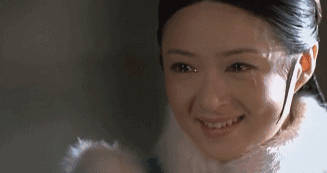# 东莞佬哭诉：月薪3500，仲未过年就已经洗咗成万蚊！“为了过这个年，你得花多少钱？”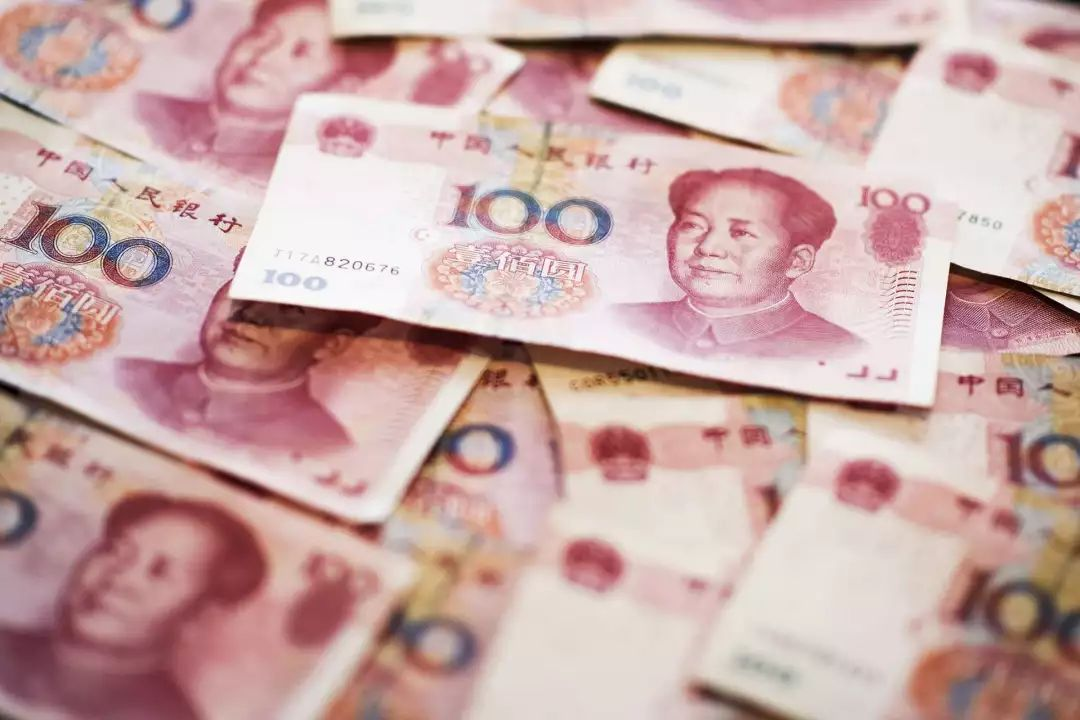1000x2+200x4=2800 or 1000x2+500x4=40001000x2+200x4=2800 or 1000x2+500x4=4000

1000x2+200x4=2800 or 1000x2+500x4=4000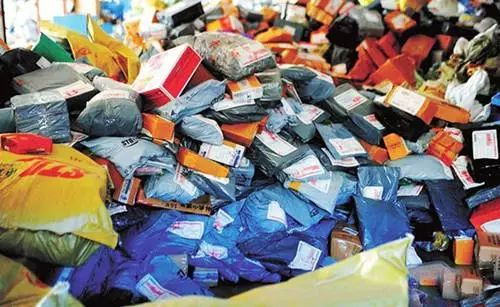500+1000+200=1700 or 500+1000+300=1800

500-700一件，你要买几件呢？谢谢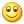600x4=2400600+600=12001000+500=15001500+500=2000 or 1500+1000=2500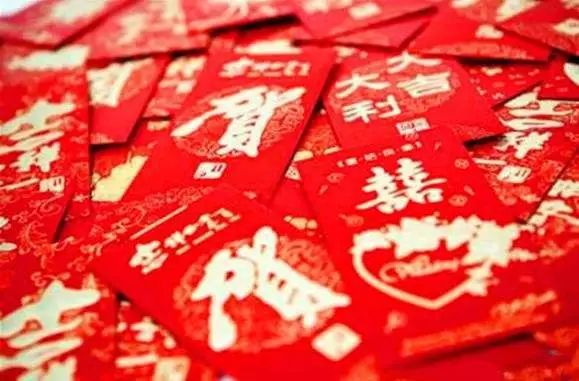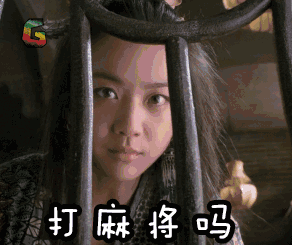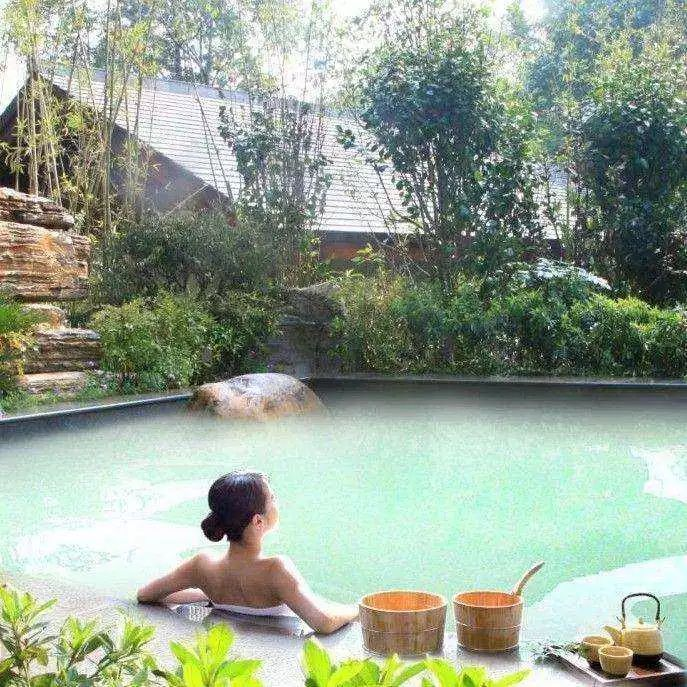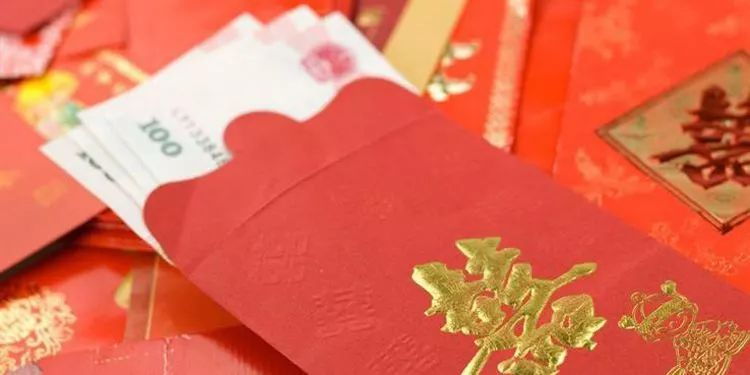2800+1700+1500+1500+500+1500=9500（最低）

8000+4200+1500+1500+1000+1500=17700（最高）

2800+1200+2500+1000=7500（最低）

4000+1200+2500+1000=8700（最高）

2800+600+1500+1000=5900（最低）

4000+600+1500+1000=7100（最高）

（几百块的鞋子我还没算进去呢）（我不想结婚并想继续上班）